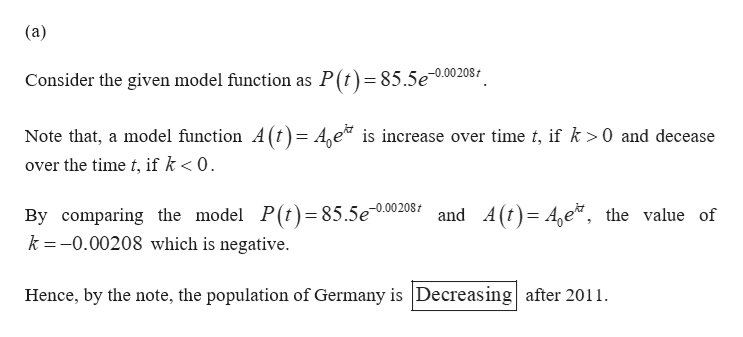# The population of Germany in 2011 was approximately 85.5 million. The model P = 85.5e^(−0.00208)t represents a short-term model for the population, t years after 2011.a. Based on this model, is the population of Germany increasing or decreasing?b. Determine the number of years after 2011 at which the population of Germany would decrease to 80 million if this trend continues. Round to the nearest year.

Question
1 views

The population of Germany in 2011 was approximately 85.5 million. The model P = 85.5e^(−0.00208)t represents a short-term model for the population, t years after 2011.

a. Based on this model, is the population of Germany increasing or decreasing?

b. Determine the number of years after 2011 at which the population of Germany would decrease to 80 million if this trend continues. Round to the nearest year.

check_circle

Step 1help_outlineImage Transcriptionclose(a) Consider the given model function as P(t)=85.5e-0.002081 Note that, a model function A (t)= A,e is increase over time t, if k >0 and decease over the time t, if k < 0. 00208 and A (t)= A,e*, the value of By comparing the model P(t)= 85.5e k =-0.00208 which is negative. Hence, by the note, the population of Germany is Decreasing after 2011. fullscreen

### Want to see the full answer?

See Solution

#### Want to see this answer and more?

Solutions are written by subject experts who are available 24/7. Questions are typically answered within 1 hour.*

See Solution
*Response times may vary by subject and question.
Tagged in

### Other